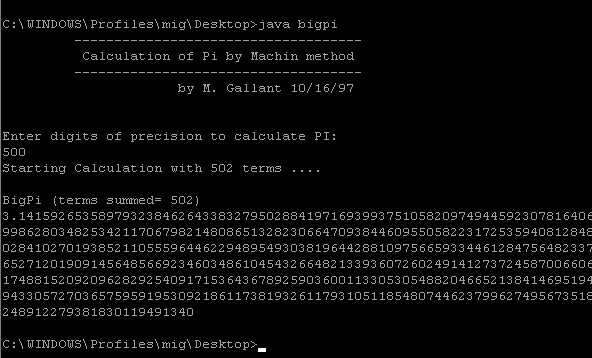Compute Pi in Java

` M. Gallant 10/17/97`

The trancendental number Pi has been calculated to billions of digits (1991 Chudnovsky brothers USA) using various algorithms based on the iterative procedures and supercomputers. One of the most significant early advances in calculating Pi was achieved by John Machin (1706) who cleverly manipulated identities based on a series for the inverse tangent function (ATN) to arrive at:

Pi = 16ATN(1/5) - 4ATN(1/239)

and combining this identity with the series definition for ATN:

ATN(x) = x - x^3/3 + x^5/5 - x^7/7 ....

one can easily calculate Pi to high precision. Since the ATN function converges in a well-behaved and predictable way, Pi can be easily calculated to extended precision provided that one has access to large math arithmetic routines. JDK1.1 provides this basic capability in the java.Math.BigDecimal class.

The image below shows the standard system-output from a standalone Java application (Java Bytecode) for calculating Pi to 500 significant digits (10 seconds on a 486dx2/66 and 1 second on a Pentium GXPro200). The user is prompted for the digits of precision to be used in the computation.References:
• D. Shanks and J. Wrench, Mathematics of Computation, 16, 1962 p.76.
• P. Beckmann, "A History of Pi", St. Martin's Press 1971.
• J. Borwein and P. Borwein, "Pi and the AGM", Wiley 1987.
• J. Borwein and P. Borwein, "Ramanuan and Pi", Sci. Am. Feb. 1988 p.112.Go Home ET